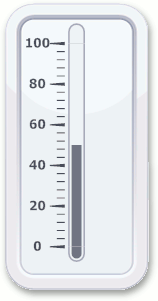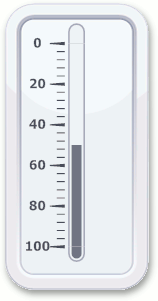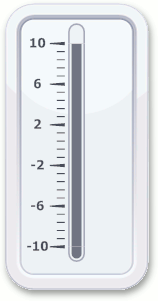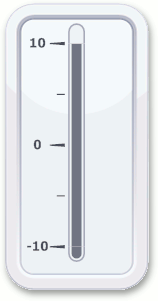21.2 (EAP/Beta)
21.1
20.2
20.1
19.2
19.1
18.2
18.1
17.2

# LinearScale.MinValue Property

Gets or sets the minimum value of the linear scale.

Namespace: DevExpress.XtraGauges.Core.Model

Assembly: DevExpress.XtraGauges.v21.1.Core.dll

## Declaration

``````[DefaultValue(0F)]
public float MinValue { get; set; }``````

## Property Value

Type Default Description
Single

0

A Single value that is the minimum limit of the linear scale.

## Remarks

The MinValue and LinearScale.MaxValue properties together define the range of values allowed for the LinearScale. The current value indicated by the level bar is specified by the LinearScale.Value property.

Note that the LinearScale.MaxValue property value shouldn’t necessarily be greater than MinValue. For instance, if you switch their values, this will simply change a linear scale’s direction.

MinValue = 0, MaxValue = 100 (Default) MinValue = 100, MaxValue = 0 (Rotated)While the MinValue and MaxValue properties define the limit values, the number of tickmarks (and therefore labels) between them is specified by the LinearScale.MinorTickCount and LinearScale.MajorTickCount properties. The following images demonstrate how these properties can be modified together to customize the linear scale.

Default

MinValue = -10, MaxValue = 10

MinValue = -10, MaxValue = 10,

MinorTickCount = 1, MajorTickCount = 3